# Buck converter - Duty cycle issue

#### onlyvinod56

Joined Oct 14, 2008
369
Hello,
I am designing a buck converter ... very basic... open loop. I am giving 12V as input and expecting the output to be changing from 0 - 12v with a duty cycle range of 10 - 90%. I have taken a circuit reference from a website.
https://electronoobs.io/uploads/tutorial_files/210/image_1_727_.png (the P-mosfet pin representation is wrong in the picture... I have corrected in my circuit).

My circuit: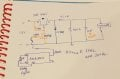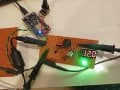I did some trials ... here are the results.

Case 1: Fs = 1KHz; The output voltage is changing from 0 - 12v with a duty cycle range of 5% to 15%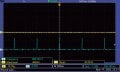o/p = 63mV; Duty Cycle = 5%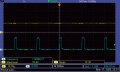o/p = 12 V; Duty Cycle = 15%

Case 2: Fs = 30KHz; The output voltage is changing from 0 - 12v with a
duty cycle range of 40% to 50%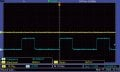o/p = 187mV; Duty Cycle = 40%o/p = 12.5V; Duty Cycle = 50%

My question is, how can I control the voltage from 0 - 12v with a duty cycle range of 10 - 90 %. Actually the circuit which I took as reference is based on ARduino controller (pulse height is 5V).... But I am working with NodeMcu(ESP8266) whose pulse height is 3.3V. Did I do any mistake with NPN gate resistor? Based on a rough calculation I got the base resistor is around 1.5 MegaOhm.

#### bertus

Joined Apr 5, 2008
21,896
Hello,

A base resistor if 1M is very large.
Do the tests again with 10K in the base line.

Bertus

•Delta Prime and ronsimpson

#### Ian0

Joined Aug 7, 2020
5,090
The output voltage is only proportional to duty cycle if the current is continuous.
For the current to be continuous the change of current over the switching cycle must be less than twice the DC current.
At 50% duty cycle, the load is 6V/500Ω = 12mA, so ΔI needs to be less than 24mA
ΔI= Vt/L
V=6V, t=16us
That requires L to be >4mH

•ronsimpson

#### crutschow

Joined Mar 14, 2008
29,777
If you look at the MOSFET base you will likely see that it is barely switching.
That MOSFET has a gate-charge of 180nC which has to be rapidly charged and discharged. so you need a very low impedance drive to the MOSFET gate (such as a push-pull driver).
Also using a newer type MOSFET with lower gate charge would also help.

And for a 30kHz operation, you need an inductor of about 10mH or greater.
You reference design uses 100mH.
How did you come up with 47µH?

If you are going to do a switching regulator, then you need to learn a little more about how to calculate the correct part values.
Random selection seldom works.

Last edited:
•Ian0

#### Ian0

Joined Aug 7, 2020
5,090
That MOSFET has a gate-charge of 180nC which has to be rapidly charged and discharged. so you need a very low impedance drive to the MOSFET gate.
Remove the transistor and its two resistors, and replace by an MCP1401 MOSFET driver.

#### onlyvinod56

Joined Oct 14, 2008
369
Hello,

A base resistor if 1M is very large.
Do the tests again with 10K in the base line.

Bertus
Changed 1M to 10K. But there is no impact of duty cycle on output. Whatever be the D, the output is equal to input.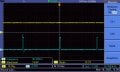Minimum Duty cycle - O/P = 12.7V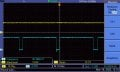Maximum Duty cycle - O/P = 12.7V

#### onlyvinod56

Joined Oct 14, 2008
369
The output voltage is only proportional to duty cycle if the current is continuous.
For the current to be continuous the change of current over the switching cycle must be less than twice the DC current.
At 50% duty cycle, the load is 6V/500Ω = 12mA, so ΔI needs to be less than 24mA
ΔI= Vt/L
V=6V, t=16us
That requires L to be >4mH
This is interesting. Why did you choose t = 16us? and the load 500 OHM is not compulsory in my case.

#### onlyvinod56

Joined Oct 14, 2008
369
If you look at the MOSFET base you will likely see that it is barely switching.
That MOSFET has a gate-charge of 180nC which has to be rapidly charged and discharged. so you need a very low impedance drive to the MOSFET gate (such as a push-pull driver).
Also using a newer type MOSFET with lower gate charge would also help.
OK. At present I dont have any other P-MOSFETs. Definitely I will try with this suggestion.
And for a 30kHz operation, you need an inductor of about 10mH or greater.
You reference design uses 100mH.
How did you come up with 47µH?
Because... I have LM2596 in my mind as it works with 33uH or 47uH.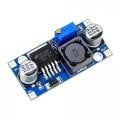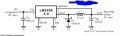I thought this 33uH or 47uH is doing the job. The only point I didnt observe is 150 KHz as per the LM2596 datasheet.

#### onlyvinod56

Joined Oct 14, 2008
369
Remove the transistor and its two resistors, and replace by an MCP1401 MOSFET driver.
I will try this later sir.

#### Ian0

Joined Aug 7, 2020
5,090
This is interesting. Why did you choose t = 16us? and the load 500 OHM is not compulsory in my case.
50% duty cycle at 30kHz.

#### Ian0

Joined Aug 7, 2020
5,090
OK. At present I dont have any other P-MOSFETs. Definitely I will try with this suggestion.

Because... I have LM2596 in my mind as it works with 33uH or 47uH.
View attachment 263089 View attachment 263090

I thought this 33uH or 47uH is doing the job. The only point I didnt observe is 150 KHz as per the LM2596 datasheet.
The higher the output current, the lower the inductance.
the higher the operating frequency, the lower the inductance.

#### onlyvinod56

Joined Oct 14, 2008
369
50% duty cycle at 30kHz.
ok ok.
So, now I will change my load. Let it be 0.5A. therefore ΔI < 1A. I will consider the current ripple be 0.5A.

ΔI = Vt/L
0.5 = (6 x t) / 40uH
t = 3.3 uSec
Period = 6.6 uSec
f = 150 KHz
the higher the operating frequency, the lower the inductance.
I will try with this 150 KHz. I cannot change my 40 uH.

#### crutschow

Joined Mar 14, 2008
29,777
The only point I didnt observe is 150 KHz as per the LM2596 datasheet.
That's a very large point when it comes to switching regulators.

It will not be easy to get a discrete circuit to operate at 150kHz.